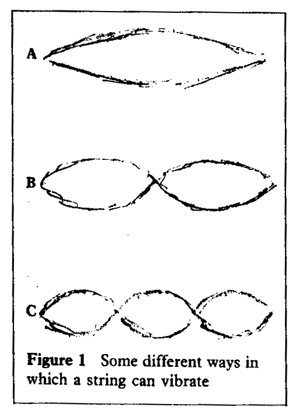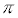[HomePage] Professor W.W. Sawyer

The Contribution of Music to Mathematical Discovery

WW Sawyer

Mathematicians, it is often said, tend to be musical. It is less well known that problems arising from music have played an important role in the discovery of fundamental mathematical ideas. Questions about the vibrations of a piano string led to a fierce controversy that forced mathematicians to clarify their ideas about area, continuity, and the convergence of series.

It is well known that there exists a mathematical theory of musical sounds. Very few people know that music has made a contribution to mathematics. In fact a suggestion made by a musician led to a total revolution in the way mathematicians approach their subject. No doubt, mathenaticians would have made this step forward sooner or later if the musician had not given this hint, but as a matter of historical record, that was how it happened.

Vibrating Strings

In the 17th century, calculus provided mathematicians with a splendid instrument for investigating gradients and velocities, and mathematicians enthusiastically set about investigating anything that had a shape or that moved. One of these topics was the vibrations of a piano string.'

Figure 1 shows some of the ways in which a string can vibrate: A produces a certain note; B produces the first harmonic, an octave higher; and C fifth higher.

In 1715, the English mathematician Brook Taylor gave this a mathematical form. If we choose a suitable unit of time and a unit of length that makes the string have length, the displacement of the string at position x and time t is given by

y = sin x cos t for vibration A
y = sin 2x cos 2t for vibration B
y = sin 3x cos 3t for vibration C

There are of course harmonics beyond these, corresponding to the numbers 4, 5, 6....

So far, these vibrations are separate possibilities. However the French composer, Rameau, said that when a note was played he heard many of these harmonics at one and the same time. In 1726 he published a theory of harmony (which I believe is still regarded as valid) based on this idea.

Mixtures of Harmonics

The Swiss mathematician, Daniel Bernouilli, picked up this hint. He formed an arbitrary mixture of all the harmonics and thus in 1753 arrived at the formula

y = a1 sin x cos t + a2 sin 2x cos 2t + a3 sin 3x cos 3t + .....

It is interesting that Bernouilli's argument is based purely on physics; there are no calculations in this part of his paper. He speaks of '
a melange of all these vibrations' and goes on to say, 'All musicians are agreed that a vibrating string gives at the same time, besides its fundamental tone, others much higher; they notice above all the mixture of the 12th and the major 17th'.

His series contains an infinite number of constants an